# 数据算法之二叉树一

## 什么是树？

• 树是计算机科学中经常用到的一种数据结构;
• 树是一种非线性的数据结构,以分层的方式存储数据;
• 树被用来存储具有层级关系的数据,比如文件系统中的文件;树还被用来存储有序列表;
• 查找、添加、删除元素性能要优于链表及数组;
• 树由一组以边连接的节点组成;

### 树的相关名词

• 父节点，子节点，兄弟节点：这个不用解释都知道吧
• 结点的度：就是分支数
• 树的度: 最大的结点度,最大也是2
• 叶子结点：没有子节点的结点
• 结点的层数：就是树有几层
• 左节点
• 右节点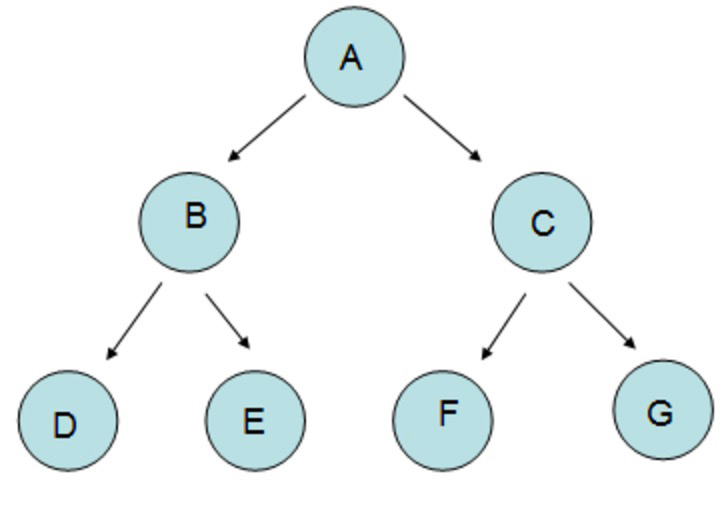### 二叉树的性质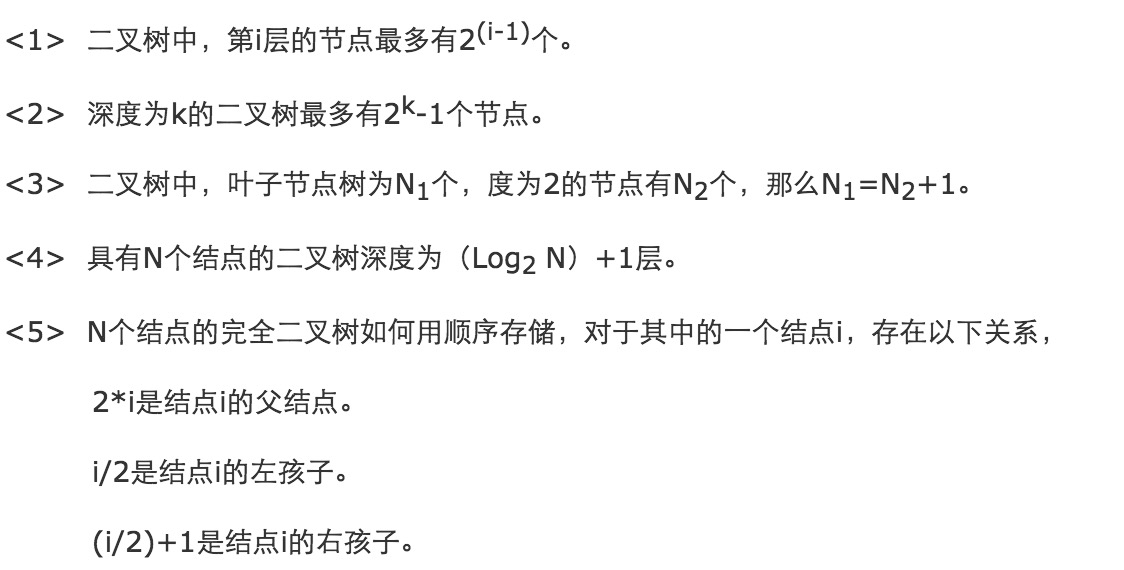## JS实现二叉树

``````function Node(data, left, right) {
this.data = data;      //用于存储数据
this.left = left;      //左节点
this.right = right;    //右节点
this.show = show;      //显示保存在节点中的数据
}

function show() {
return this.data;
}
``````

``````function BST() {
this.root = null;       //用于表示根节点，并初始化为null
this.insert = insert;   //插入节点的方法

this.preOrder = preOrder; //先序遍历
this.inOrder = inOrder; //中序遍历
this.postOrder = postOrder; //后序遍历

this.getMin = getMin;
this.getMax = getMax;
this.find = find;

this.remove = remove;         //简单地接受待删除数据
this.removeNode = removeNode; //删除
}
``````

1. 判断有无根节点，没有的话，插入到根节点;
2. 如果待插入节点保存的数据小于当前节点，则设新的当前节点为原节点的左节点; 反之，执行第 4 步;
3. 如果当前节点的左节点为null，就将新的节点插入这个位置，退出循环; 反之，继续执行下一次循环;
4. 设新的当前节点为原节点的右节点;
5. 如果当前节点的右节点为null，就将新的节点插入这个位置，退出循环; 反之，继续执行下一次循环;
``````
function insert(data) {
var n = new Node(data, null, null),
current,
parent;

if (this.root == null) {
this.root = n;
} else {
current = this.root;   //根节点指向当前节点
while(true) {
parent = current;  //根节点定义为父节点
if (data < current.data) {
current = current.left;
if (current == null) {
parent.left = n;
break;
}
} else {
current = current.right;
if (current == null) {
parent.right = n;
break;
}
}
}
}
}

``````

### 遍历的顺序

``````先序：根节点==>左子树==>右子树

``````

``````function inOrder(node) {
if (!(node == null)) {
inOrder(node.left);
console.log(node.show() + " ");
inOrder(node.right);
}
}
``````

``````var nums = new BST();
nums.insert(23);
nums.insert(45);
nums.insert(16);
nums.insert(37);
nums.insert(3);
nums.insert(99);
nums.insert(22);
console.log(inOrder(nums.root));
``````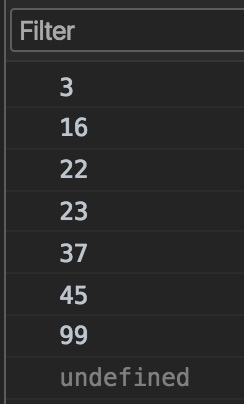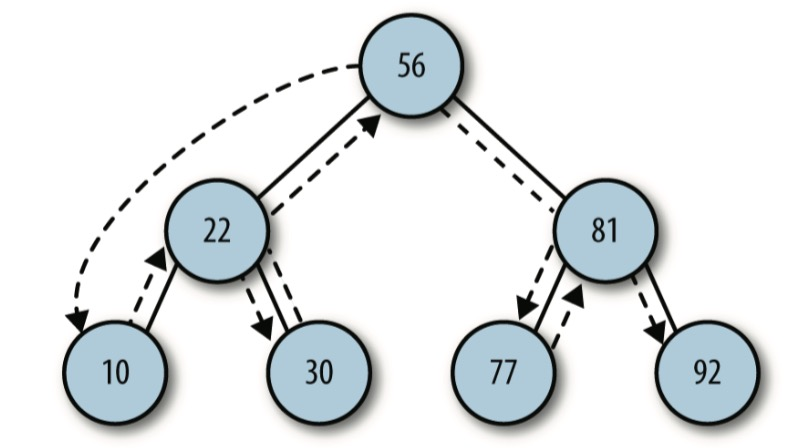``````function preOrder(node) {
if (!(node == null)) {
console.log(node.show() + " ");
preOrder(node.left);
preOrder(node.right);
}
}
``````

inOrder() 和 preOrder() 方法的唯一区别,就是 if 语句中代码的顺序。在 inOrder() 方法中,show() 函数像三明治一样夹在两个递归调用之间;在 preOrder() 方法中,show() 函数放在两个递归调用之前。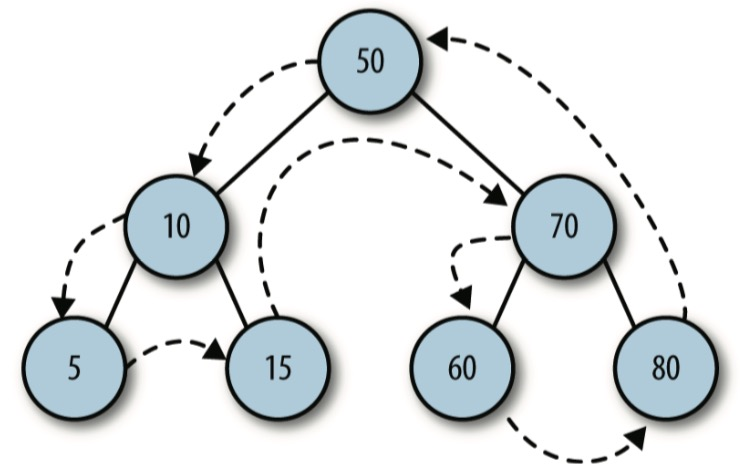``````function postOrder(node) {
if (!(node == null)) {
postOrder(node.left);
console.log(node.right);
putstr(node.show() + " ");
}
}
``````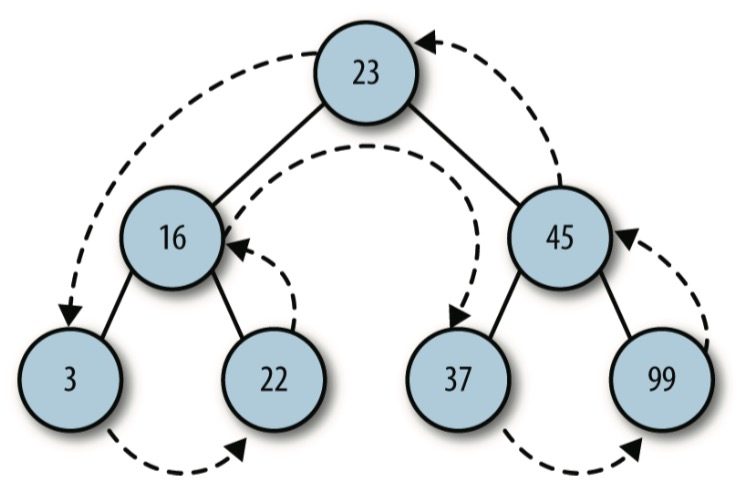### 二叉树的查找

• 查找给定值;
• 查找最小值;
• 查找最大值。

``````function getMax() {
var current = this.root;
while (!(current.right == null)) {
current = current.right;
}
return current.data;
}
``````

``````function getMin() {
var current = this.root;
while (!(current.left == null)) {
current = current.left;
}
return current.data;
}
``````

``````var nums = new BST();
nums.insert(23);
nums.insert(45);
nums.insert(16);
nums.insert(37);
nums.insert(3);
nums.insert(99);
nums.insert(22);

var min = nums.getMin();
print("minimum value: " + min);
print("\n");
var max = nums.getMax();
print("maximum value: " + max);
``````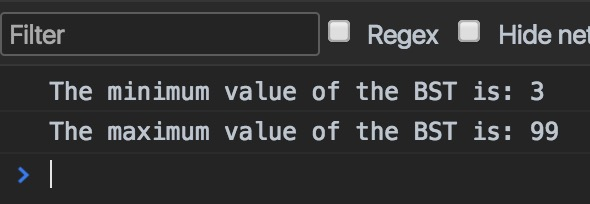``````function find(data) {
var current = this.root;
while (current != null) {
if (current.data == data) {
return current;
}  else if (data < current.data) {
current = current.left;
} else {
current = current.right;
}
}
return null;
}
``````